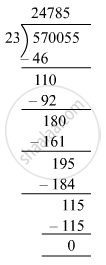# The cost price of 23 TV sets is Rs 570055. Find the cost of each such set. - Mathematics

Sum

The cost price of 23 TV sets is Rs 570055. Find the cost of each such set.

#### Solution

Cost price of 23 TV sets = Rs 5,70,055
Cost price of 1 TV set = 570055 ÷ 23∴ The cost of one TV set is Rs 24,785.

Concept: Concept for Whole Numbers
Is there an error in this question or solution?

#### APPEARS IN

RS Aggarwal Class 6 Mathematics
Chapter 3 Whole Numbers
Test Paper 3 | Q 7 | Page 59